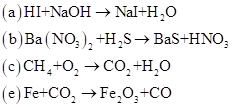# 4-17 Balance each equation.### Introduction to General, Organic a...

11th Edition
Frederick A. Bettelheim + 4 others
Publisher: Cengage Learning
ISBN: 9781285869759### Introduction to General, Organic a...

11th Edition
Frederick A. Bettelheim + 4 others
Publisher: Cengage Learning
ISBN: 9781285869759

#### Solutions

Chapter 4, Problem 4.17P
Textbook Problem

## 4-17 Balance each equation.Expert Solution

(a)

Interpretation Introduction

Interpretation:

The given chemical equation should be balanced.

Concept Introduction:

For balancing the reaction, number of moles of reactant should be equivalent to number of moles of product.

Balancing of equation takes place by adding number of moles or stochiometric coefficient before the reactant and product so to balance the number of atoms present in the molecule.

For the reaction:

A +  B   AB2

2 moles of B are required to balance the reaction as follows:

A + 2 B   AB2

The balanced chemical equation is

HI + NaOH   NaI + H2O

### Explanation of Solution

1 mol of HI reacts with 1 mol NaOH to produce 1 mole of NaI with 1 mol of water.

The balanced chemical equation is

HI + NaOH   NaI + H2O

Now, the reaction is balanced with 1 number of I on each side that is reactant and product.

The reaction is balanced with 1 number of oxygen atom on each side that is reactant and product.

The reaction is balanced with 2 number of hydrogen atoms on each side that is reactant and product.

Thus, the reaction is already balanced.

Expert Solution

(b)

Interpretation Introduction

Interpretation:

The given chemical equation should be balanced.

Concept Introduction:

For balancing the reaction, number of moles of reactant should be equivalent to number of moles of product.

Balancing of equation takes place by adding number of moles or stochiometric coefficient before the reactant and product so to balance the number of atoms present in the molecule.

For the reaction:

A +  B   AB2

2 moles of B are required to balance the reaction as follows:

A + 2 B   AB2

The balanced chemical equation is

Ba( NO3)2+ H2S  BaS + 2 HNO3

### Explanation of Solution

The given chemical reaction is as follows:

Ba( NO3)2+ H2S  BaS + HNO3

Give coefficient 2 to HNO3 to balance the number of nitrogen and oxygen atoms.

Ba( NO3)2+ H2S  BaS + 2HNO3

Thus, the balanced chemical reaction is as follows:

Ba( NO3)2+ H2S  BaS + 2HNO3

Expert Solution

(c)

Interpretation Introduction

Interpretation:

The given chemical equation should be balanced.

Concept Introduction:

For balancing the reaction, number of moles of reactant should be equivalent to number of moles of product.

Balancing of equation takes place by adding number of moles or stochiometric coefficient before the reactant and product so to balance the number of atoms present in the molecule.

For the reaction:

A +  B   AB2

2 moles of B are required to balance the reaction as follows:

A + 2 B   AB2

The balanced chemical equation is

CH4+ 2O2 CO2+ 2H2O

### Explanation of Solution

The given chemical reaction is as follows:

CH4+ O2 CO2+ H2O

To balance the number of hydrogen atoms, give coefficient 2 to H2O .

CH4+ O2 CO2+ 2H2O

Now, give coefficient 2 to  O2 to balance the number of oxygen atoms.

CH4+ 2O2 CO2+ 2H2O

Thus, the balanced chemical reaction is as follows:

CH4+ 2O2 CO2+ 2H2O

Expert Solution

(d)

Interpretation Introduction

Interpretation:

The given chemical equation should be balanced.

Concept Introduction:

For balancing the reaction, number of moles of reactant should be equivalent to number of moles of product.

Balancing of equation takes place by adding number of moles or stochiometric coefficient before the reactant and product so to balance the number of atoms present in the molecule.

For the reaction:

A +  B   AB2

2 moles of B are required to balance the reaction as follows:

A + 2 B   AB2

The balanced chemical equation is

2C4H1013O2 8CO2+ 10H2O

### Explanation of Solution

The given chemical equation is as follows:

C4H10+ O2 CO2+ H2O

Give coefficient 4 to CO2 to balance the number of carbon atoms.

C4H10+ O2 4CO2+ H2O

Now, balance the hydrogen atoms by giving coefficient 5 to H2O

C4H10+ O2 4CO2+ 5H2O

To balance the number of oxygen atoms give coefficient132 to O2 on the reactant side.

C4H10132O2 4CO2+ 5H2O

Or,

2C4H1013O2 8CO2+ 10H2O

Thus, it is the balanced chemical reaction.

Expert Solution

(e)

Interpretation Introduction

Interpretation:

The given chemical equation should be balanced.

Concept Introduction:

For balancing the reaction, number of moles of reactant should be equivalent to number of moles of product.

Balancing of equation takes place by adding number of moles or stochiometric coefficient before the reactant and product so to balance the number of atoms present in the molecule.

For the reaction:

A +  B   AB2

2 moles of B are required to balance the reaction as follows:

A + 2 B   AB2

The balanced chemical equation is

2Fe +3CO2   Fe2O3+3CO

### Explanation of Solution

The given reaction is as follows:

Fe +CO2   Fe2O3+CO

Give coefficient 2 to Fe in the reactant side to balance the number of Fe atoms.

2Fe +CO2   Fe2O3+CO

Now, to balance the number of oxygen atoms, give coefficient 3 to CO2 on reactant side and 3 to CO on product side as follows:

2Fe +3CO2   Fe2O3+3CO

Thus, the balanced chemical reaction will be:

2Fe +3CO2   Fe2O3+3CO

### Want to see more full solutions like this?

Subscribe now to access step-by-step solutions to millions of textbook problems written by subject matter experts!

### Want to see more full solutions like this?

Subscribe now to access step-by-step solutions to millions of textbook problems written by subject matter experts!